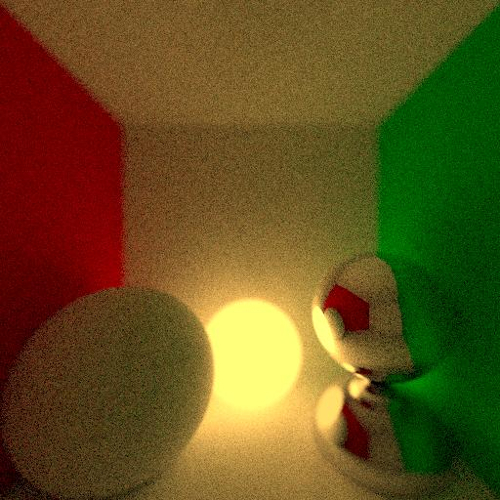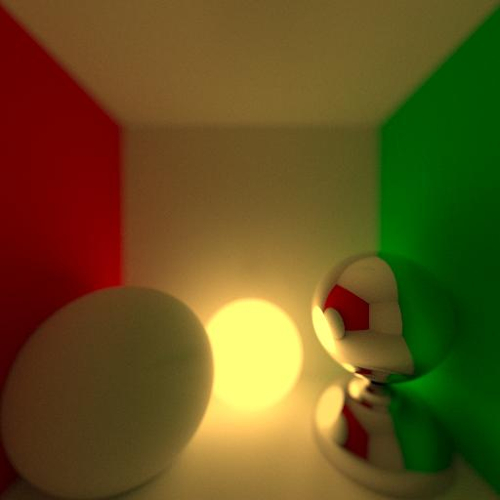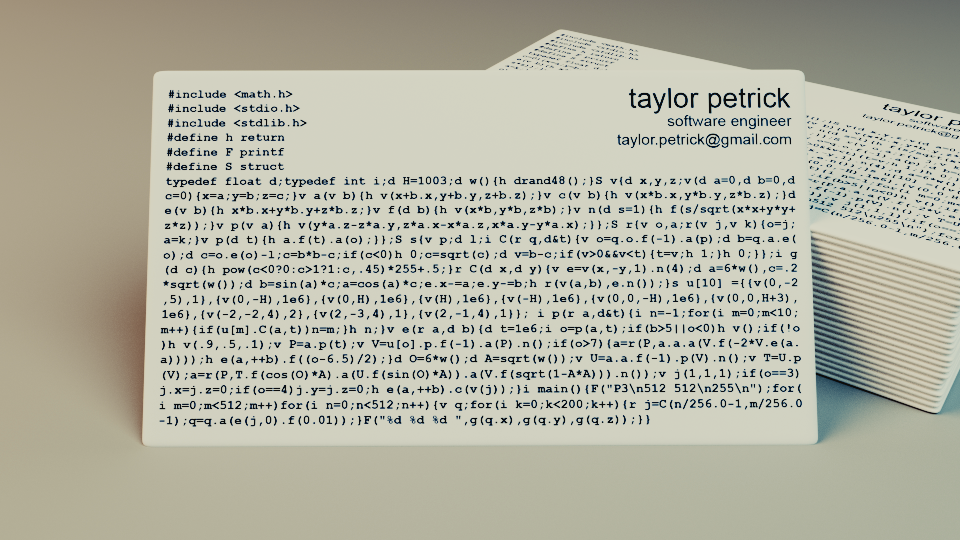# The Challenge

There’s a challenge amongst graphics programmers that has been floating around for many years. The gist of it is to write a ray tracer that is small enough to print on a business card, but still valid code that can compile, run and produce some sort of image as output. Its intended to be an exercise that involves a strong understanding of ray tracing, as well as skills with writing minified code. I believe the first reference to it was in the book Graphics Gems IV, though I can’t say for sure.

In North America, business cards are usually around 3.5 inches by 2 inches. I took a look a few printing companies and they tend to support at least 350 dpi in terms of print quality. Based on that I figured that an implementation would have to be at most 24 lines of 120 characters to printable. Fewer lines is of course better, as the font size of the code can be increased when printing the card.

# My Implementation

I don’t really use business cards, however the challenge is interesting so I decided to give it a try. The following code is my crack at a business card ray tracer. I ended up using 86 character lines to properly fit my code to a business card aspect ratio.

```#include <math.h>
#include <stdio.h>
#include <stdlib.h>
#define h return
#define F printf
#define S struct
typedef float d;typedef int i;d H=1003;d w(){h drand48();}S v{d x,y,z;v(d a=0,d b=0,d
c=0){x=a;y=b;z=c;}v a(v b){h v(x+b.x,y+b.y,z+b.z);}v c(v b){h v(x*b.x,y*b.y,z*b.z);}d
e(v b){h x*b.x+y*b.y+z*b.z;}v f(d b){h v(x*b,y*b,z*b);}v n(d s=1){h f(s/sqrt(x*x+y*y+
z*z));}v p(v a){h v(y*a.z-z*a.y,z*a.x-x*a.z,x*a.y-y*a.x);}};S r{v o,a;r(v j,v k){o=j;
a=k;}v p(d t){h a.f(t).a(o);}};S s{v p;d l;i C(r q,d&t){v o=q.o.f(-1).a(p);d b=q.a.e(
o);d c=o.e(o)-l;c=b*b-c;if(c<0)h 0;c=sqrt(c);d v=b-c;if(v>0&&v<t){t=v;h 1;}h 0;}};i g
(d c){h pow(c<0?0:c>1?1:c,.45)*255+.5;}r C(d x,d y){v e=v(x,-y,1).n(4);d a=6*w(),c=.2
*sqrt(w());d b=sin(a)*c;a=cos(a)*c;e.x-=a;e.y-=b;h r(v(a,b),e.n());}s u ={{v(0,-2
,5),1},{v(0,-H),1e6},{v(0,H),1e6},{v(H),1e6},{v(-H),1e6},{v(0,0,-H),1e6},{v(0,0,H+3),
1e6},{v(-2,-2,4),2},{v(2,-3,4),1},{v(2,-1,4),1}}; i p(r a,d&t){i n=-1;for(i m=0;m<10;
m++){if(u[m].C(a,t))n=m;}h n;}v e(r a,d b){d t=1e6;i o=p(a,t);if(b>5||o<0)h v();if(!o
)h v(.9,.5,.1);v P=a.p(t);v V=u[o].p.f(-1).a(P).n();if(o>7){a=r(P,a.a.a(V.f(-2*V.e(a.
a))));h e(a,++b).f((o-6.5)/2);}d O=6*w();d A=sqrt(w());v U=a.a.f(-1).p(V).n();v T=U.p
(V);a=r(P,T.f(cos(O)*A).a(U.f(sin(O)*A)).a(V.f(sqrt(1-A*A))).n());v j(1,1,1);if(o==3)
j.x=j.z=0;if(o==4)j.y=j.z=0;h e(a,++b).c(v(j));}i main(){F("P3\n512 512\n255\n");for(
i m=0;m<512;m++)for(i n=0;n<512;n++){v q;for(i k=0;k<100;k++){r j=C(n/256.0-1,m/256.0
-1);q=q.a(e(j,0).f(0.02));}F("%d %d %d ",g(q.x),g(q.y),g(q.z));}}
```

The code can be compiled on Linux as follows:

`\$ g++ -O3 business-rt.cpp`

The O3 compiler optimization flag is pretty important or else the program will take a terribly long time to complete. The program writes a .ppm image to standard output, so generally you’d want to run it by redirecting output to a file:

`\$ ./a.out > output.ppm`

When run as-is, it takes about 45 seconds to produce the image on the left. It’s also possible to increase the samples/pixel without really affecting the overall code size, though it does make the run time slower since the code isn’t optimized for speed. The image on the right was rendered using 2,000 samples/pixel.100 and 2,000 sample renders

I’m planning on doing a write up on the design and optimization of the code at a later date, so I’ll hold off on implementation details for now. At a high level, the code is a path tracer with full global illumination and depth of field. Its able to handle both diffuse and reflective surfaces, as well as a single light source. All objects in the scene are spheres, including the “planes” that form the walls of the box. By making spheres with extremely large radii and placing them far from the origin, it’s possible to emulate planes without the complexity of supporting plane primitive shapes.

The scene itself is a bit like the Cornell Box and is intended to show off the features of the ray tracer. A large diffuse sphere was placed near the red wall to emphasize global illumination effects. The colored tint on the surface of that sphere is caused by light reflecting off the wall. All of the objects create soft shadows since no direct light source sampling is done. The blurring due to the depth of field is highly exaggerated, but I liked the effect so I ended up keeping it. It’s possible to tweak the DOF a bit by adjusting the focal length and aperture radius. The final output image has it’s brightness ramped up and is then run through a gamma filter before output, causing the bloom/overexposure effect.

One of the interesting aspects of the renders is that there’s heavy lense distortion near the edges of the images. This is especially noticable on the ball on the left, which looks more like an egg/ellipsoid than a perfect sphere. The reason for the distortion is that the camera has a very wide field of view. This wasn’t ideal, however with that FOV configuration that math worked out in a way that helped decrease code size — the z-component for the unnormalized camera rays reduced to a value of 1.0.

That fact that it’s possible to write a ray tracer with such a small amount of code is indicative of how simple the core algorithm actually is. In this case the code is a mess that’s impossible to read, but using standard coding conventions no more than a few hundred lines are needed for a fairly robust path tracer. The complexity of a practical implementation mainly lies in the acceleration structures, threading and need to support different samplers and surface types.